## Python Tutorial

Python HOME Python Intro Python Get Started Python Syntax Python Comments Python Variables Python Data Types Python Numbers Python Casting Python Strings Python Booleans Python Operators Python Lists Python Tuples Python Sets Python Dictionaries Python If...Else Python While Loops Python For Loops Python Functions Python Lambda Python Arrays Python Classes/Objects Python Inheritance Python Iterators Python Polymorphism Python Scope Python Modules Python Dates Python Math Python JSON Python RegEx Python PIP Python Try...Except Python User Input Python String Formatting

## File Handling

Python File Handling Python Read Files Python Write/Create Files Python Delete Files

## Python Modules

NumPy Tutorial Pandas Tutorial SciPy Tutorial Django Tutorial

## Python Matplotlib

Matplotlib Intro Matplotlib Get Started Matplotlib Pyplot Matplotlib Plotting Matplotlib Markers Matplotlib Line Matplotlib Labels Matplotlib Grid Matplotlib Subplot Matplotlib Scatter Matplotlib Bars Matplotlib Histograms Matplotlib Pie Charts

## Machine Learning

Getting Started Mean Median Mode Standard Deviation Percentile Data Distribution Normal Data Distribution Scatter Plot Linear Regression Polynomial Regression Multiple Regression Scale Train/Test Decision Tree Confusion Matrix Hierarchical Clustering Logistic Regression Grid Search Categorical Data K-means Bootstrap Aggregation Cross Validation AUC - ROC Curve K-nearest neighbors

## Python MySQL

MySQL Get Started MySQL Create Database MySQL Create Table MySQL Insert MySQL Select MySQL Where MySQL Order By MySQL Delete MySQL Drop Table MySQL Update MySQL Limit MySQL Join

## Python MongoDB

MongoDB Get Started MongoDB Create Database MongoDB Create Collection MongoDB Insert MongoDB Find MongoDB Query MongoDB Sort MongoDB Delete MongoDB Drop Collection MongoDB Update MongoDB Limit

## Python Reference

Python Overview Python Built-in Functions Python String Methods Python List Methods Python Dictionary Methods Python Tuple Methods Python Set Methods Python File Methods Python Keywords Python Exceptions Python Glossary

## Module Reference

Random Module Requests Module Statistics Module Math Module cMath Module

## Python How To

Remove List Duplicates Reverse a String Add Two Numbers

## Python Examples

Python Examples Python Compiler Python Exercises Python Quiz Python Bootcamp Python Certificate

# Matplotlib Markers

## Markers

You can use the keyword argument `marker` to emphasize each point with a specified marker:

### Example

Mark each point with a circle:

import matplotlib.pyplot as plt
import numpy as np

ypoints = np.array([3, 8, 1, 10])

plt.plot(ypoints, marker = 'o')
plt.show()

### Result: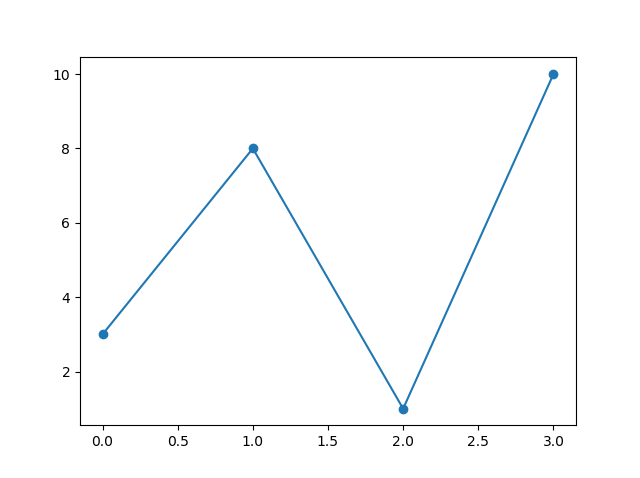Try it Yourself »

### Example

Mark each point with a star:

...
plt.plot(ypoints, marker = '*')
...

### Result: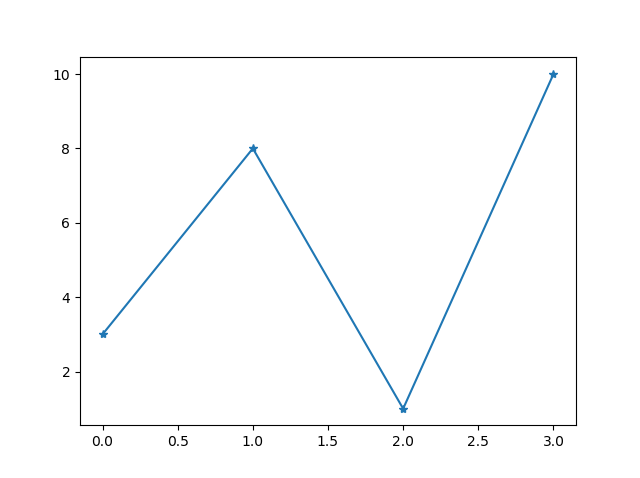Try it Yourself »

## Marker Reference

You can choose any of these markers:

Marker Description
'o' Circle Try it »
'*' Star Try it »
'.' Point Try it »
',' Pixel Try it »
'x' X Try it »
'X' X (filled) Try it »
'+' Plus Try it »
'P' Plus (filled) Try it »
's' Square Try it »
'D' Diamond Try it »
'd' Diamond (thin) Try it »
'p' Pentagon Try it »
'H' Hexagon Try it »
'h' Hexagon Try it »
'v' Triangle Down Try it »
'^' Triangle Up Try it »
'<' Triangle Left Try it »
'>' Triangle Right Try it »
'1' Tri Down Try it »
'2' Tri Up Try it »
'3' Tri Left Try it »
'4' Tri Right Try it »
'|' Vline Try it »
'_' Hline Try it »

## Format Strings `fmt`

You can use also use the shortcut string notation parameter to specify the marker.

This parameter is also called `fmt`, and is written with this syntax:

`marker|line|color`

### Example

Mark each point with a circle:

import matplotlib.pyplot as plt
import numpy as np

ypoints = np.array([3, 8, 1, 10])

plt.plot(ypoints, 'o:r')
plt.show()

### Result: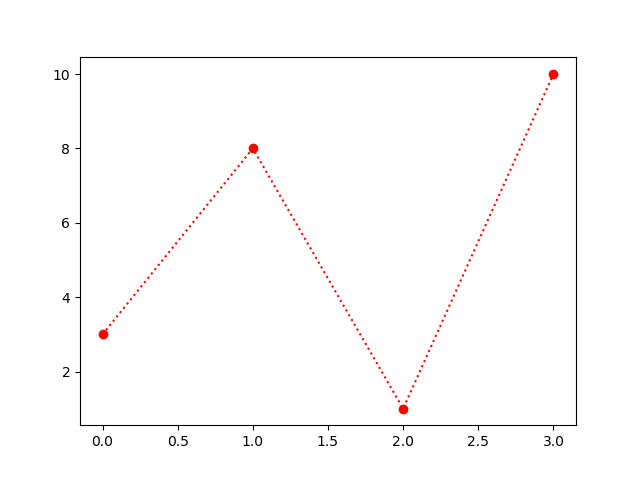Try it Yourself »

The marker value can be anything from the Marker Reference above.

The line value can be one of the following:

## Line Reference

Line Syntax Description
'-' Solid line Try it »
':' Dotted line Try it »
'--' Dashed line Try it »
'-.' Dashed/dotted line Try it »

Note: If you leave out the line value in the fmt parameter, no line will be plotted.

The short color value can be one of the following:

## Color Reference

Color Syntax Description
'r' Red Try it »
'g' Green Try it »
'b' Blue Try it »
'c' Cyan Try it »
'm' Magenta Try it »
'y' Yellow Try it »
'k' Black Try it »
'w' White Try it »

## Marker Size

You can use the keyword argument `markersize` or the shorter version, `ms` to set the size of the markers:

### Example

Set the size of the markers to 20:

import matplotlib.pyplot as plt
import numpy as np

ypoints = np.array([3, 8, 1, 10])

plt.plot(ypoints, marker = 'o', ms = 20)
plt.show()

### Result: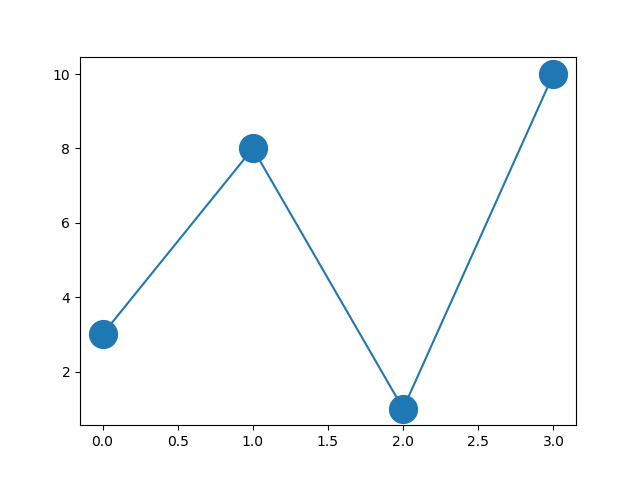Try it Yourself »

## Marker Color

You can use the keyword argument `markeredgecolor` or the shorter `mec` to set the color of the edge of the markers:

### Example

Set the EDGE color to red:

import matplotlib.pyplot as plt
import numpy as np

ypoints = np.array([3, 8, 1, 10])

plt.plot(ypoints, marker = 'o', ms = 20, mec = 'r')
plt.show()

### Result: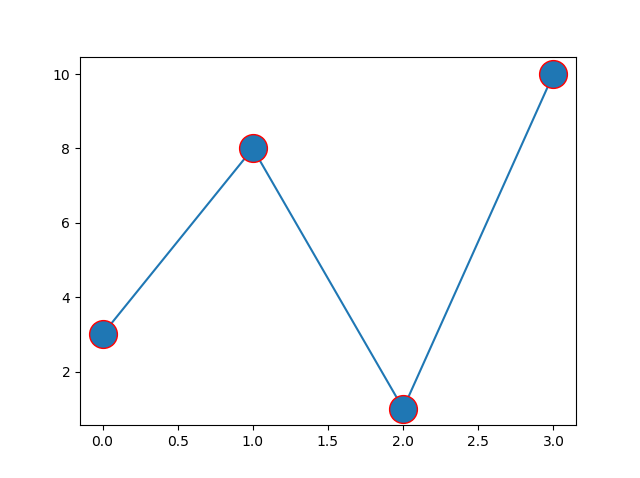Try it Yourself »

You can use the keyword argument `markerfacecolor` or the shorter `mfc` to set the color inside the edge of the markers:

### Example

Set the FACE color to red:

import matplotlib.pyplot as plt
import numpy as np

ypoints = np.array([3, 8, 1, 10])

plt.plot(ypoints, marker = 'o', ms = 20, mfc = 'r')
plt.show()

### Result: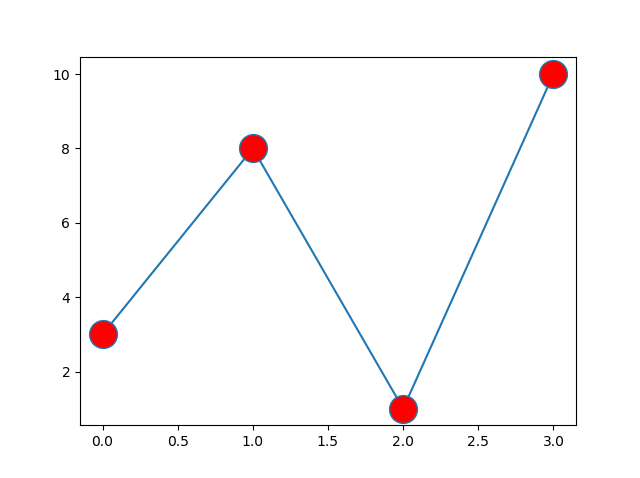Try it Yourself »

Use both the `mec` and `mfc` arguments to color the entire marker:

### Example

Set the color of both the edge and the face to red:

import matplotlib.pyplot as plt
import numpy as np

ypoints = np.array([3, 8, 1, 10])

plt.plot(ypoints, marker = 'o', ms = 20, mec = 'r', mfc = 'r')
plt.show()

### Result: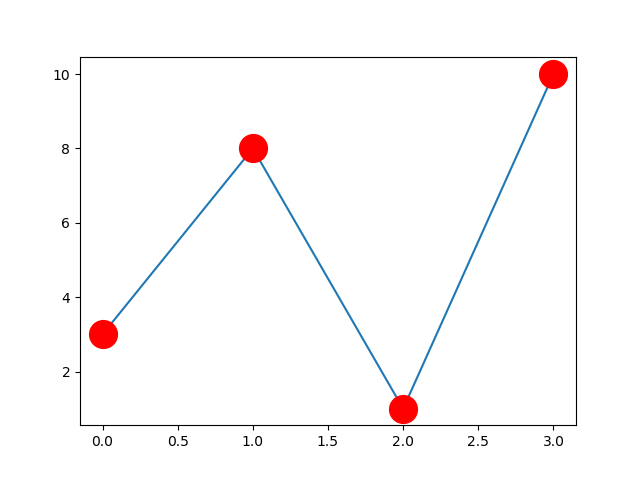Try it Yourself »

You can also use Hexadecimal color values:

### Example

Mark each point with a beautiful green color:

...
plt.plot(ypoints, marker = 'o', ms = 20, mec = '#4CAF50', mfc = '#4CAF50')
...

### Result: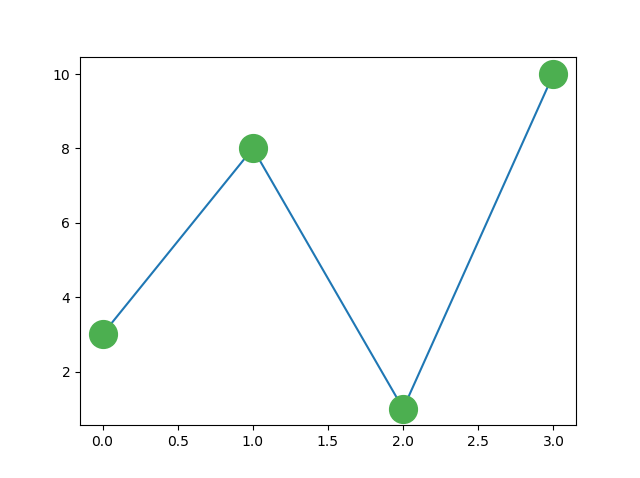Try it Yourself »

Or any of the 140 supported color names.

### Example

Mark each point with the color named "hotpink":

...
plt.plot(ypoints, marker = 'o', ms = 20, mec = 'hotpink', mfc = 'hotpink')
...

### Result: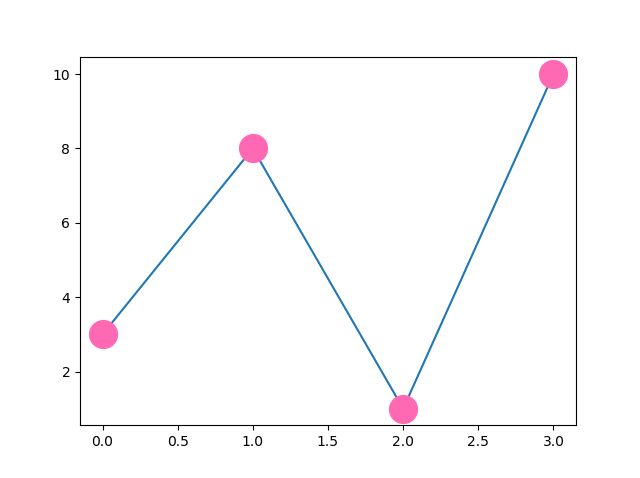Try it Yourself »